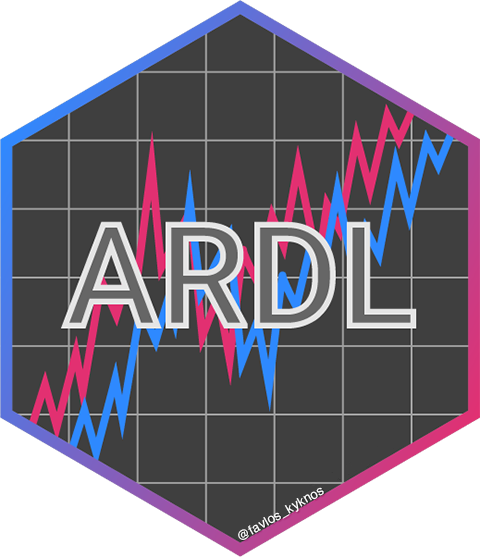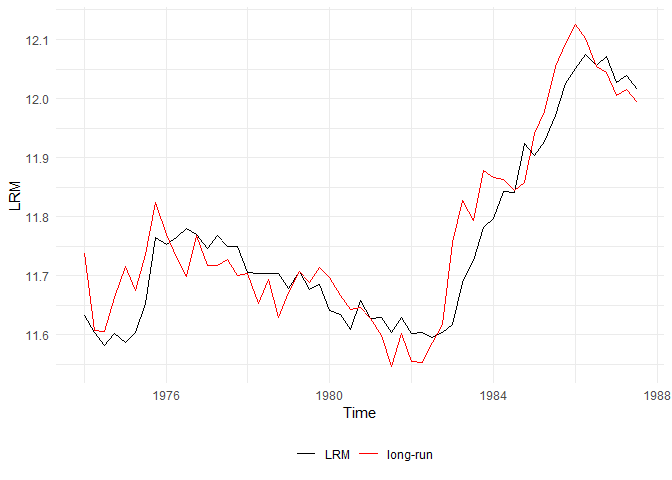# ARDL## Overview

`ARDL` creates complex autoregressive distributed lag (ARDL) models providing just the order and automatically constructs the underlying unrestricted and restricted error correction model (ECM). It also performs the bounds-test for cointegration as described in Pesaran et al. (2001) and provides the multipliers and the cointegrating equation.

## Why `ARDL`?

• Estimate complex ARDL models just providing the ARDL order
• Estimate the conditional ECM just providing the underlying ARDL model or the order
• Estimate the long-run multipliers
• Apply the bound test for no cointegration (Pesaran et al., 2001)
• Both the F-test and the t-test are available
• The p-value is also available along with the critical value bounds for specific level of statistical significance
• Exact p-values and critical value bounds are available, along with the asymptotic ones

## Installation

``````# You can install the released version of ARDL from CRAN:
install.packages("ARDL")

# Or the latest development version from GitHub:
install.packages("devtools")
devtools::install_github("Natsiopoulos/ARDL")``````

## Usage

This is a basic example which shows how to use the main functions of the `ARDL` package.

Assume that we want to model the `LRM` (logarithm of real money, M2) as a function of `LRY`, `IBO` and `IDE` (see `?denmark`). The problem is that applying an OLS regression on non-stationary data would result into a spurious regression. The estimated parameters would be consistent only if the series were cointegrated.

``````library(ARDL)
data(denmark)``````

First, we find the best ARDL specification. We search up to order 5.

``````models <- auto_ardl(LRM ~ LRY + IBO + IDE, data = denmark, max_order = 5)
#> Warning: The `x` argument of `as_tibble.matrix()` must have unique column names if `.name_repair` is omitted as of tibble 2.0.0.
#> Using compatibility `.name_repair`.
#> This warning is displayed once every 8 hours.
#> Call `lifecycle::last_warnings()` to see where this warning was generated.

# The top 20 models according to the AIC
models\$top_orders
#>    LRM LRY IBO IDE       AIC
#> 1    3   1   3   2 -251.0259
#> 2    3   1   3   3 -250.1144
#> 3    2   2   0   0 -249.6266
#> 4    3   2   3   2 -249.1087
#> 5    3   2   3   3 -248.1858
#> 6    2   2   0   1 -247.7786
#> 7    2   1   0   0 -247.5643
#> 8    2   2   1   1 -246.6885
#> 9    3   3   3   3 -246.3061
#> 10   2   2   1   2 -246.2709
#> 11   2   1   1   1 -245.8736
#> 12   2   2   2   2 -245.7722
#> 13   1   1   0   0 -245.6620
#> 14   2   1   2   2 -245.1712
#> 15   3   1   2   2 -245.0996
#> 16   1   0   0   0 -244.4317
#> 17   1   1   0   1 -243.7702
#> 18   5   5   5   5 -243.3120
#> 19   4   1   3   2 -243.0728
#> 20   4   1   3   3 -242.4378

# The best model was found to be the ARDL(3,1,3,2)
ardl_3132 <- models\$best_model
ardl_3132\$order
#>  3 1 3 2
summary(ardl_3132)
#>
#> Time series regression with "zooreg" data:
#> Start = 1974 Q4, End = 1987 Q3
#>
#> Call:
#> dynlm::dynlm(formula = full_formula, data = data, start = start,
#>     end = end)
#>
#> Residuals:
#>       Min        1Q    Median        3Q       Max
#> -0.029939 -0.008856 -0.002562  0.008190  0.072577
#>
#> Coefficients:
#>             Estimate Std. Error t value Pr(>|t|)
#> (Intercept)   2.6202     0.5678   4.615 4.19e-05 ***
#> L(LRM, 1)     0.3192     0.1367   2.336 0.024735 *
#> L(LRM, 2)     0.5326     0.1324   4.024 0.000255 ***
#> L(LRM, 3)    -0.2687     0.1021  -2.631 0.012143 *
#> LRY           0.6728     0.1312   5.129 8.32e-06 ***
#> L(LRY, 1)    -0.2574     0.1472  -1.749 0.088146 .
#> IBO          -1.0785     0.3217  -3.353 0.001790 **
#> L(IBO, 1)    -0.1062     0.5858  -0.181 0.857081
#> L(IBO, 2)     0.2877     0.5691   0.505 0.616067
#> L(IBO, 3)    -0.9947     0.3925  -2.534 0.015401 *
#> IDE           0.1255     0.5545   0.226 0.822161
#> L(IDE, 1)    -0.3280     0.7213  -0.455 0.651847
#> L(IDE, 2)     1.4079     0.5520   2.550 0.014803 *
#> ---
#> Signif. codes:  0 '***' 0.001 '**' 0.01 '*' 0.05 '.' 0.1 ' ' 1
#>
#> Residual standard error: 0.0191 on 39 degrees of freedom
#> Multiple R-squared:  0.988,  Adjusted R-squared:  0.9843
#> F-statistic: 266.8 on 12 and 39 DF,  p-value: < 2.2e-16``````

Then we can estimate the UECM (Unrestricted Error Correction Model) of the underlying ARDL(3,1,3,2).

``````uecm_3132 <- uecm(ardl_3132)
summary(uecm_3132)
#>
#> Time series regression with "zooreg" data:
#> Start = 1974 Q4, End = 1987 Q3
#>
#> Call:
#> dynlm::dynlm(formula = full_formula, data = data, start = start,
#>     end = end)
#>
#> Residuals:
#>       Min        1Q    Median        3Q       Max
#> -0.029939 -0.008856 -0.002562  0.008190  0.072577
#>
#> Coefficients:
#>              Estimate Std. Error t value Pr(>|t|)
#> (Intercept)   2.62019    0.56777   4.615 4.19e-05 ***
#> L(LRM, 1)    -0.41685    0.09166  -4.548 5.15e-05 ***
#> L(LRY, 1)     0.41538    0.11761   3.532  0.00108 **
#> L(IBO, 1)    -1.89172    0.39111  -4.837 2.09e-05 ***
#> L(IDE, 1)     1.20534    0.44690   2.697  0.01028 *
#> d(L(LRM, 1)) -0.26394    0.10192  -2.590  0.01343 *
#> d(L(LRM, 2))  0.26867    0.10213   2.631  0.01214 *
#> d(LRY)        0.67280    0.13116   5.129 8.32e-06 ***
#> d(IBO)       -1.07852    0.32170  -3.353  0.00179 **
#> d(L(IBO, 1))  0.70701    0.46874   1.508  0.13953
#> d(L(IBO, 2))  0.99468    0.39251   2.534  0.01540 *
#> d(IDE)        0.12546    0.55445   0.226  0.82216
#> d(L(IDE, 1)) -1.40786    0.55204  -2.550  0.01480 *
#> ---
#> Signif. codes:  0 '***' 0.001 '**' 0.01 '*' 0.05 '.' 0.1 ' ' 1
#>
#> Residual standard error: 0.0191 on 39 degrees of freedom
#> Multiple R-squared:  0.7458, Adjusted R-squared:  0.6676
#> F-statistic: 9.537 on 12 and 39 DF,  p-value: 3.001e-08``````

And also the RECM (Restricted Error Correction Model) of the underlying ARDL(3,1,3,2), allowing the constant to join the short-run relationship (case 2), instead of the long-run (case 3).

``````recm_3132 <- recm(uecm_3132, case = 2)
summary(recm_3132)
#>
#> Time series regression with "zooreg" data:
#> Start = 1974 Q4, End = 1987 Q3
#>
#> Call:
#> dynlm::dynlm(formula = full_formula, data = data, start = start,
#>     end = end)
#>
#> Residuals:
#>       Min        1Q    Median        3Q       Max
#> -0.029939 -0.008856 -0.002562  0.008190  0.072577
#>
#> Coefficients:
#>              Estimate Std. Error t value Pr(>|t|)
#> d(L(LRM, 1)) -0.26394    0.09008  -2.930 0.005405 **
#> d(L(LRM, 2))  0.26867    0.09127   2.944 0.005214 **
#> d(LRY)        0.67280    0.11591   5.805 7.03e-07 ***
#> d(IBO)       -1.07852    0.30025  -3.592 0.000837 ***
#> d(L(IBO, 1))  0.70701    0.44359   1.594 0.118300
#> d(L(IBO, 2))  0.99468    0.36491   2.726 0.009242 **
#> d(IDE)        0.12546    0.48290   0.260 0.796248
#> d(L(IDE, 1)) -1.40786    0.48867  -2.881 0.006160 **
#> ect          -0.41685    0.07849  -5.311 3.63e-06 ***
#> ---
#> Signif. codes:  0 '***' 0.001 '**' 0.01 '*' 0.05 '.' 0.1 ' ' 1
#>
#> Residual standard error: 0.01819 on 43 degrees of freedom
#>   (0 observations deleted due to missingness)
#> Multiple R-squared:  0.7613, Adjusted R-squared:  0.7113
#> F-statistic: 15.24 on 9 and 43 DF,  p-value: 9.545e-11``````

Let’s test if there is a long-run levels relationship (cointegration) using the bounds test from Pesaran et al. (2001).

``````# The bounds F-test (under the case 2) rejects the NULL hypothesis (let's say, assuming alpha = 0.01) with p-value = 0.004418.
bounds_f_test(ardl_3132, case = 2)
#>
#>  Bounds F-test (Wald) for no cointegration
#>
#> data:  d(LRM) ~ L(LRM, 1) + L(LRY, 1) + L(IBO, 1) + L(IDE, 1) + d(L(LRM,     1)) + d(L(LRM, 2)) + d(LRY) + d(IBO) + d(L(IBO, 1)) + d(L(IBO,     2)) + d(IDE) + d(L(IDE, 1))
#> F = 5.1168, p-value = 0.004418
#> alternative hypothesis: Possible cointegration
#> null values:
#>    k    T
#>    3 1000

# The bounds t-test (under the case 3) rejects the NULL hypothesis (let's say, assuming alpha = 0.01) with p-value = 0.005538.
# We also provide the critical value bounds for alpha = 0.01.
tbounds <- bounds_t_test(uecm_3132, case = 3, alpha = 0.01)
tbounds
#>
#>  Bounds t-test for no cointegration
#>
#> data:  d(LRM) ~ L(LRM, 1) + L(LRY, 1) + L(IBO, 1) + L(IDE, 1) + d(L(LRM,     1)) + d(L(LRM, 2)) + d(LRY) + d(IBO) + d(L(IBO, 1)) + d(L(IBO,     2)) + d(IDE) + d(L(IDE, 1))
#> t = -4.5479, Lower-bound I(0) = -3.43, Upper-bound I(1) = -4.37,
#> p-value = 0.005538
#> alternative hypothesis: Possible cointegration
#> null values:
#>    k    T
#>    3 1000

# Here is a more clear view of the main results.
tbounds\$tab
#>   statistic lower.bound upper.bound alpha     p.value
#> t -4.547939       -3.43       -4.37  0.01 0.005538316``````

Here we have the short-run and the long-run multipliers (with standard errors, t-statistics and p-values).

``````multipliers(ardl_3132, type = "sr")
#>          term   estimate std.error t.statistic      p.value
#> 1 (Intercept)  6.2856579 0.7719160   8.1429302 6.107445e-10
#> 2         LRY  1.6139988 0.1239310  13.0233660 8.809795e-16
#> 3         IBO -2.5872899 0.5202961  -4.9727264 1.364936e-05
#> 4         IDE  0.3009803 0.9950853   0.3024668 7.639036e-01
multipliers(ardl_3132)
#>          term   estimate std.error t.statistic      p.value
#> 1 (Intercept)  6.2856579 0.7719160    8.142930 6.107445e-10
#> 2         LRY  0.9964676 0.1239310    8.040503 8.358472e-10
#> 3         IBO -4.5381160 0.5202961   -8.722180 1.058619e-10
#> 4         IDE  2.8915201 0.9950853    2.905801 6.009239e-03``````

Now let’s graphically check the estimated long-run relationship (cointegrating equation) against the dependent variable `LRM`.

``ce <- coint_eq(ardl_3132, case = 2)``
``````library(zoo) # for cbind.zoo
library(xts) # for xts

den <- cbind.zoo(LRM = denmark[,"LRM"], ce)
den <- xts(den)

# make the plot
den <- xts(den)
plot(den, legend.loc = "right")``````## Ease of use

Let’s see what it takes to build the above ARDL(3,1,3,2) model.

Using the `ARDL` package (literally one line of code):

``ardl_model <- ardl(LRM ~ LRY + IBO + IDE, data = denmark, order = c(3,1,3,2))``

Without the `ARDL` package:
(Using the `dynlm` package, because striving with the `lm` function would require extra data transformation to behave like time-series)

``````library(dynlm)

dynlm_ardl_model <- dynlm(LRM ~ L(LRM, 1) + L(LRM, 2) + L(LRM, 3) + LRY + L(LRY, 1) +
IBO + L(IBO, 1) + L(IBO, 2) + L(IBO, 3) +
IDE + L(IDE, 1) + L(IDE, 2), data = denmark)``````
``````identical(ardl_model\$coefficients, dynlm_ardl_model\$coefficients)
#>  TRUE``````

An ARDL model has a relatively simple structure, although the difference in typing effort is noticeable.

Not to mention the complex transformation for an ECM. The extra typing is the least of your problems trying to do this. First you would need to figure out the exact structure of the model!

Using the `ARDL` package (literally one line of code):

``uecm_model <- uecm(ardl_model)``

Without the `ARDL` package:

``````dynlm_uecm_model <- dynlm(d(LRM) ~ L(LRM, 1) + L(LRY, 1) + L(IBO, 1) +
L(IDE, 1) + d(L(LRM, 1)) + d(L(LRM, 2)) +
d(LRY) + d(IBO) + d(L(IBO, 1)) + d(L(IBO, 2)) +
d(IDE) + d(L(IDE, 1)), data = denmark)``````
``````identical(uecm_model\$coefficients, dynlm_uecm_model\$coefficients)
#>  TRUE``````

Pesaran, M. H., Shin, Y., & Smith, R. J. (2001). Bounds testing approaches to the analysis of level relationships. Journal of Applied Econometrics, 16(3), 289-326